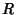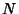Weyl Tensor

The Tensorwhereis the Riemann Tensor andis the Curvature Scalar. The Weyl tensor is defined so that every Contraction between indices gives 0. In particular,. The number of independent components for a Weyl tensor in-D is given by